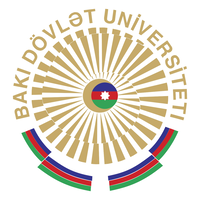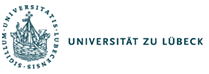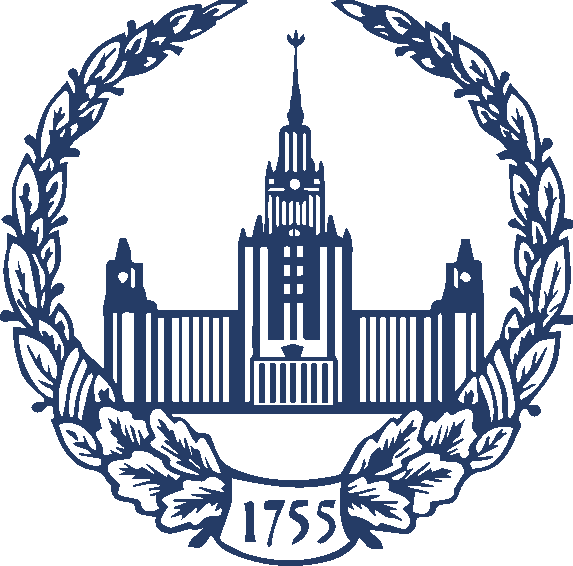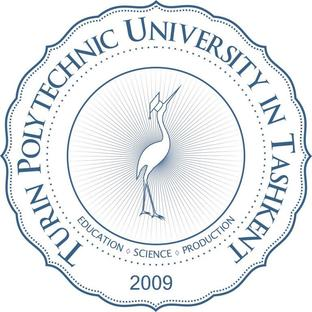﻿ Partnership Members – sompaty.eu

## Members## #Baku## #Baku## #Baku## #Baku## #Baku## #Baku## #Baku

Beneficiaries

Technische Universität Ilmenau, Ilmenau, Germany

• will PT symmetry and applications to metamaterials,
• quantum graphs in terms of extension theory,
• perturbation and spectral theory
• perturbation theory for DAEs and applications to the optimization of integrated circuits

, Czech Republic
will

• quantum graph models and PT symmetric operators in combination with metamaterials,
• singular Schrödinger and Dirac operators,
• leaky quantum structures
• dissipative and/or many-body effects in graphs and optical fibres.
• spectral theory
• inverse problems and
• differential equations.

Universität zu Lübeck, Lübeck, Germany
will

• all data-driven approaches for the numerical solution of the operator equations,
• fast algorithms for different approximation methods and stability,
• convergence and localization results,
• non-linear and adaptive variants for all approximation theoretical results.
Partners

Institute of Mathematics and Mathematical ModellingAlmaty, Kazakhstan

• non-local boundary value problems for hyperbolic integro-differential equations
• nonlocal boundary value problems.
• Qualitative properties of boundary value problems for parabolic integro-differential equations

Institute of Applied Mathematics, Baku State University, Baku, Azerbaijan

•  Inverse Scattering problems
• Inverse boundary value problems for equations with partial derivatives
• a discrete Rosser’s model for the movement of a gasliquid mixture in oil production

Turin Polytechnic University TashkentTashkent, Uzbekistan

• Linear and nonlinear PDEs on metric graphs
• PT-symmetry and soliton solutions
• Numerical solutions of PDEs on branched domains